Theory and Modern Applications

# Symmetry Properties of Higher-Order Bernoulli Polynomials

## Abstract

We investigate properties of identities and some interesting identities of symmetry for the Bernoulli polynomials of higher order using the multivariate-adic invariant integral on.

## 1. Introduction

Letbe a fixed prime number. Throughout this paperandwill, respectively, denote the ring of-adic rational integers, the field of-adic rational numbers, and the completion of algebraic closure of. For, we use the notation. Letbe the space of uniformly differentiable functions onand letbe the normalized exponential valuation ofwith. Forwith, the-Volkenborn integral onis defined as(1.1)

(see [1, 2]). The ordinary-adic invariant integral onis given by(1.2)

(see ). Let. Then we easily see that(1.3)

From (1.3), we can derive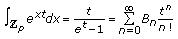(1.4)

(see [2, 810]), whereare theth Bernoulli numbers.

By (1.2) and (1.3), we easily see that(1.5)

whereforIt is known that the Bernoulli polynomials are defined by(1.6)

whereare called theth Bernoulli polynomials. The Bernoulli polynomials of order, denoted, are defined as(1.7)

(see ). Then the values ofatare called the Bernoulli numbers of order. When, the polynomials or numbers are called the Bernoulli polynomials or numbers. The purpose of this paper is to investigate some interesting properties of symmetry for the multivariate-adic invariant integral on. From the properties of symmetry for the multivariate-adic invariant integral on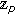, we derive some interesting identities of symmetry for the Bernoulli polynomials of higher order.

## 2. Symmetry Properties of Higher-Order Bernoulli Polynomials

Let. Then we define(2.1)

From (2.1), we note that(2.2)

whereIn (2.1), we note thatis symmetric in. By (2.1), we see that(2.3)

It is easy to see that(2.4)

From (2.1), (2.3), and the above formula, we can derive(2.5)

By the symmetry ofinand, we see that(2.6)

By comparing the coefficients on both sides of (2.5) and (2.6), we obtain the following theorem.

Theorem 2.1.

For, one has(2.7)

Let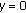andin (2.7). Then we have the following corollary.

Corollary 2.2.

For, one has(2.8)

If we take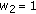in (2.8), then we also obtain the following corollary.

Corollary 2.3.

For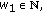one has(2.9)

By the definition of, we easily see that(2.10)

From the symmetric property ofin, we note that(2.11)

By comparing the coefficients on both sides of (2.10) and (2.11), we obtain the following theorem.

Theorem 2.4.

For, one has(2.12)

Letandin (2.12). Then we obtain the following Corollary 2.5.

Corollary 2.5.

For, one has(2.13)

From (2.12), we can get the well-known result due to Raabe:(2.14)

## References

1. Kim T: Symmetry -adic invariant integral on for Bernoulli and Euler polynomials. Journal of Difference Equations and Applications 2008,14(12):1267–1277. 10.1080/10236190801943220

2. Kim T: On a -analogue of the -adic log gamma functions and related integrals. Journal of Number Theory 1999,76(2):320–329. 10.1006/jnth.1999.2373

3. Abramowitz M, Stegun IA: Handbook of Mathematical Functions. National Bureau of Standards; 1964.

4. Jordan Ch: Calculus of Finite Differences. 2nd edition. Chelsea, New York, NY, USA; 1950.

5. Milne-Thomson LM: The Calculus of Finite Differences. Macmillan, London, UK; 1933.

6. Nörlund NE: Vorlesungen über Differenzenrechnung. Springer, Berlin, Germany; 1924.

7. Kim YH: On the -adic interpolation functions of the generalized twisted -Euler numbers. International Journal of Mathematical Analysis 2009, 3: 897–904.

8. Kim T: -Volkenborn integration. Russian Journal of Mathematical Physics 2002,9(3):288–299.

9. Kim T: -Bernoulli numbers and polynomials associated with Gaussian binomial coefficients. Russian Journal of Mathematical Physics 2008,15(1):51–57.

10. Kim T: Analytic continuation of multiple -zeta functions and their values at negative integers. Russian Journal of Mathematical Physics 2004,11(1):71–76.

11. Kim T: Non-Archimedean -integrals associated with multiple Changhee -Bernoulli polynomials. Russian Journal of Mathematical Physics 2003,10(1):91–98.

12. Kim T: Symmetry of power sum polynomials and multivariate fermionic -adic invariant integral on . Russian Journal of Mathematical Physics 2009,16(1):93–96. 10.1134/S1061920809010063

13. Ozden H, Simsek Y: A new extension of -Euler numbers and polynomials related to their interpolation functions. Applied Mathematics Letters 2008,21(9):934–939. 10.1016/j.aml.2007.10.005

14. Simsek Y: On -adic twisted --functions related to generalized twisted Bernoulli numbers. Russian Journal of Mathematical Physics 2006,13(3):340–348. 10.1134/S1061920806030095

15. Kim Y-H, Hwang K-W: A symmetry of power sum and twisted Bernoulli polynomials. Advanced Studies in Contemporary Mathematics 2009,18(2):127–133.

## Author information

Authors

### Corresponding authors

Correspondence to Taekyun Kim or Kyung-Won Hwang.

## Rights and permissions

Reprints and Permissions

Kim, T., Hwang, KW. & Kim, YH. Symmetry Properties of Higher-Order Bernoulli Polynomials. Adv Differ Equ 2009, 318639 (2009). https://doi.org/10.1155/2009/318639

• Revised:

• Accepted:

• Published:

• DOI: https://doi.org/10.1155/2009/318639

### Keywords

• Differential Equation
• Partial Differential Equation
• Ordinary Differential Equation
• Functional Analysis
• Functional Equation